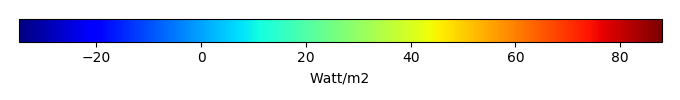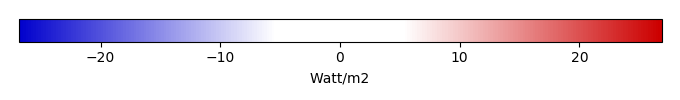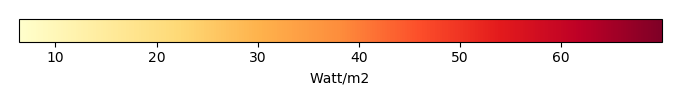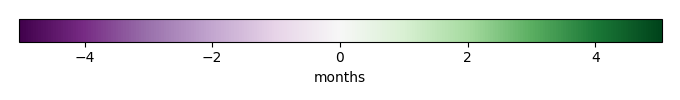# Mean State

Period Mean (original grids) [Watt/m2]
Model Period Mean (intersection) [Watt/m2]
Model Period Mean (complement) [Watt/m2]
Benchmark Period Mean (intersection) [Watt/m2]
Benchmark Period Mean (complement) [Watt/m2]
Bias [Watt/m2]
RMSE [Watt/m2]
Phase Shift [months]
Bias Score 
RMSE Score 
Seasonal Cycle Score 
Spatial Distribution Score 
Overall Score 
Benchmark [-] 44.1
CLM5PHSOFF [-] 44.7 43.8 53.4 44.2 12.4 -0.488 15.8 1.57 0.45 0.23 0.75 0.93 0.52
CLM5PHSON [-] 45.4 45.0 52.0 44.2 12.4 1.09 14.3 1.87 0.48 0.27 0.70 0.95 0.53
Period Mean (original grids) [Watt/m2]
Model Period Mean (intersection) [Watt/m2]
Model Period Mean (complement) [Watt/m2]
Benchmark Period Mean (intersection) [Watt/m2]
Benchmark Period Mean (complement) [Watt/m2]
Bias [Watt/m2]
RMSE [Watt/m2]
Phase Shift [months]
Bias Score 
RMSE Score 
Seasonal Cycle Score 
Spatial Distribution Score 
Overall Score 
Benchmark [-] 57.5
CLM5PHSOFF [-] 49.8 49.7 76.1 57.5 20.8 -8.01 19.9 0.569 0.43 0.44 0.96 0.86 0.62
CLM5PHSON [-] 50.8 50.9 67.0 57.5 20.8 -6.70 20.0 0.772 0.45 0.43 0.94 0.84 0.62
Period Mean (original grids) [Watt/m2]
Model Period Mean (intersection) [Watt/m2]
Model Period Mean (complement) [Watt/m2]
Benchmark Period Mean (intersection) [Watt/m2]
Benchmark Period Mean (complement) [Watt/m2]
Bias [Watt/m2]
RMSE [Watt/m2]
Phase Shift [months]
Bias Score 
RMSE Score 
Seasonal Cycle Score 
Spatial Distribution Score 
Overall Score 
Benchmark [-] 52.8
CLM5PHSOFF [-] 56.3 55.2 66.4 53.0 19.6 2.15 18.1 0.474 0.58 0.46 0.95 0.97 0.68
CLM5PHSON [-] 56.3 56.1 61.4 53.0 19.6 3.43 17.7 0.747 0.58 0.49 0.91 0.97 0.69
Period Mean (original grids) [Watt/m2]
Model Period Mean (intersection) [Watt/m2]
Model Period Mean (complement) [Watt/m2]
Benchmark Period Mean (intersection) [Watt/m2]
Benchmark Period Mean (complement) [Watt/m2]
Bias [Watt/m2]
RMSE [Watt/m2]
Phase Shift [months]
Bias Score 
RMSE Score 
Seasonal Cycle Score 
Spatial Distribution Score 
Overall Score 
Benchmark [-] 18.0
CLM5PHSOFF [-] -11.0 15.4 4.83 18.1 5.64 -2.93 21.7 0.726 0.77 0.46 0.94 0.92 0.71
CLM5PHSON [-] 15.6 16.0 7.19 18.1 5.64 -2.63 19.1 0.548 0.79 0.51 0.96 0.93 0.74
Period Mean (original grids) [Watt/m2]
Model Period Mean (intersection) [Watt/m2]
Model Period Mean (complement) [Watt/m2]
Benchmark Period Mean (intersection) [Watt/m2]
Benchmark Period Mean (complement) [Watt/m2]
Bias [Watt/m2]
RMSE [Watt/m2]
Phase Shift [months]
Bias Score 
RMSE Score 
Seasonal Cycle Score 
Spatial Distribution Score 
Overall Score 
Benchmark [-] 22.1
CLM5PHSOFF [-] 28.4 25.9 33.2 22.8 10.8 3.21 15.4 1.47 0.26 0.19 0.78 0.79 0.44
CLM5PHSON [-] 32.3 31.3 36.9 22.8 10.8 10.5 16.4 1.34 0.31 0.20 0.80 0.87 0.47
Period Mean (original grids) [Watt/m2]
Model Period Mean (intersection) [Watt/m2]
Model Period Mean (complement) [Watt/m2]
Benchmark Period Mean (intersection) [Watt/m2]
Benchmark Period Mean (complement) [Watt/m2]
Bias [Watt/m2]
RMSE [Watt/m2]
Phase Shift [months]
Bias Score 
RMSE Score 
Seasonal Cycle Score 
Spatial Distribution Score 
Overall Score 
Benchmark [-] 27.0
CLM5PHSOFF [-] 19.9 19.7 29.7 27.1 8.81 -7.36 18.0 0.673 0.70 0.56 0.95 0.97 0.75
CLM5PHSON [-] 20.6 20.5 27.0 27.1 8.81 -6.35 15.9 0.553 0.73 0.60 0.95 0.93 0.76
Period Mean (original grids) [Watt/m2]
Model Period Mean (intersection) [Watt/m2]
Model Period Mean (complement) [Watt/m2]
Benchmark Period Mean (intersection) [Watt/m2]
Benchmark Period Mean (complement) [Watt/m2]
Bias [Watt/m2]
RMSE [Watt/m2]
Phase Shift [months]
Bias Score 
RMSE Score 
Seasonal Cycle Score 
Spatial Distribution Score 
Overall Score 
Benchmark [-] 28.4
CLM5PHSOFF [-] 36.9 36.6 39.6 28.4 24.4 8.11 18.8 0.716 0.67 0.53 0.93 0.94 0.72
CLM5PHSON [-] 35.5 35.2 39.6 28.4 24.4 6.80 19.3 0.795 0.66 0.52 0.92 0.94 0.71
Period Mean (original grids) [Watt/m2]
Model Period Mean (intersection) [Watt/m2]
Model Period Mean (complement) [Watt/m2]
Benchmark Period Mean (intersection) [Watt/m2]
Benchmark Period Mean (complement) [Watt/m2]
Bias [Watt/m2]
RMSE [Watt/m2]
Phase Shift [months]
Bias Score 
RMSE Score 
Seasonal Cycle Score 
Spatial Distribution Score 
Overall Score 
Benchmark [-] 40.3
CLM5PHSOFF [-] 39.6 39.1 43.2 40.6 11.0 -1.62 18.8 0.606 0.54 0.49 0.95 0.86 0.67
CLM5PHSON [-] 37.6 37.1 42.1 40.6 11.0 -3.33 20.5 0.882 0.50 0.46 0.91 0.88 0.64
Period Mean (original grids) [Watt/m2]
Model Period Mean (intersection) [Watt/m2]
Model Period Mean (complement) [Watt/m2]
Benchmark Period Mean (intersection) [Watt/m2]
Benchmark Period Mean (complement) [Watt/m2]
Bias [Watt/m2]
RMSE [Watt/m2]
Phase Shift [months]
Bias Score 
RMSE Score 
Seasonal Cycle Score 
Spatial Distribution Score 
Overall Score 
Benchmark [-] 15.2
CLM5PHSOFF [-] 5.54 6.38 -2.54 15.2 3.78 -8.87 21.5 0.928 0.69 0.48 0.93 0.90 0.69
CLM5PHSON [-] 4.37 4.77 -2.26 15.2 3.78 -10.7 22.1 0.816 0.64 0.49 0.93 0.85 0.68
Period Mean (original grids) [Watt/m2]
Model Period Mean (intersection) [Watt/m2]
Model Period Mean (complement) [Watt/m2]
Benchmark Period Mean (intersection) [Watt/m2]
Benchmark Period Mean (complement) [Watt/m2]
Bias [Watt/m2]
RMSE [Watt/m2]
Phase Shift [months]
Bias Score 
RMSE Score 
Seasonal Cycle Score 
Spatial Distribution Score 
Overall Score 
Benchmark [-] 56.5
CLM5PHSOFF [-] 59.2 59.8 54.4 56.9 15.0 2.78 17.4 0.642 0.70 0.57 0.95 0.81 0.72
CLM5PHSON [-] 59.7 60.1 51.0 56.9 15.0 2.71 15.4 0.540 0.71 0.62 0.96 0.89 0.76
Period Mean (original grids) [Watt/m2]
Model Period Mean (intersection) [Watt/m2]
Model Period Mean (complement) [Watt/m2]
Benchmark Period Mean (intersection) [Watt/m2]
Benchmark Period Mean (complement) [Watt/m2]
Bias [Watt/m2]
RMSE [Watt/m2]
Phase Shift [months]
Bias Score 
RMSE Score 
Seasonal Cycle Score 
Spatial Distribution Score 
Overall Score 
Benchmark [-] 20.2
CLM5PHSOFF [-] 26.1 24.5 36.6 20.4 7.08 4.04 16.7 0.654 0.72 0.60 0.94 0.80 0.73
CLM5PHSON [-] 19.6 19.2 29.0 20.4 7.08 -0.160 16.0 0.832 0.72 0.60 0.92 0.82 0.73
Period Mean (original grids) [Watt/m2]
Model Period Mean (intersection) [Watt/m2]
Model Period Mean (complement) [Watt/m2]
Benchmark Period Mean (intersection) [Watt/m2]
Benchmark Period Mean (complement) [Watt/m2]
Bias [Watt/m2]
RMSE [Watt/m2]
Phase Shift [months]
Bias Score 
RMSE Score 
Seasonal Cycle Score 
Spatial Distribution Score 
Overall Score 
Benchmark [-] 34.3
CLM5PHSOFF [-] 35.6 36.9 13.9 34.6 11.5 2.22 19.7 0.823 0.59 0.48 0.92 0.93 0.68
CLM5PHSON [-] 31.0 36.8 6.87 34.6 11.5 2.16 19.1 0.881 0.60 0.49 0.91 0.92 0.68
Period Mean (original grids) [Watt/m2]
Model Period Mean (intersection) [Watt/m2]
Model Period Mean (complement) [Watt/m2]
Benchmark Period Mean (intersection) [Watt/m2]
Benchmark Period Mean (complement) [Watt/m2]
Bias [Watt/m2]
RMSE [Watt/m2]
Phase Shift [months]
Bias Score 
RMSE Score 
Seasonal Cycle Score 
Spatial Distribution Score 
Overall Score 
Benchmark [-] 22.7
CLM5PHSOFF [-] 47.7 49.9 45.4 22.8 18.3 26.9 36.3 1.27 0.52 0.51 0.93 0.78 0.65
CLM5PHSON [-] 45.5 48.2 43.0 22.8 18.3 26.2 36.6 1.26 0.51 0.49 0.94 0.78 0.64
Period Mean (original grids) [Watt/m2]
Model Period Mean (intersection) [Watt/m2]
Model Period Mean (complement) [Watt/m2]
Benchmark Period Mean (intersection) [Watt/m2]
Benchmark Period Mean (complement) [Watt/m2]
Bias [Watt/m2]
RMSE [Watt/m2]
Phase Shift [months]
Bias Score 
RMSE Score 
Seasonal Cycle Score 
Spatial Distribution Score 
Overall Score 
Benchmark [-] 17.0
CLM5PHSOFF [-] 8.63 9.84 0.308 17.0 4.51 -7.27 21.7 1.03 0.72 0.49 0.91 0.90 0.70
CLM5PHSON [-] 9.34 9.87 0.733 17.0 4.51 -7.63 21.0 0.966 0.71 0.51 0.91 0.88 0.70
Period Mean (original grids) [Watt/m2]
Model Period Mean (intersection) [Watt/m2]
Model Period Mean (complement) [Watt/m2]
Benchmark Period Mean (intersection) [Watt/m2]
Benchmark Period Mean (complement) [Watt/m2]
Bias [Watt/m2]
RMSE [Watt/m2]
Phase Shift [months]
Bias Score 
RMSE Score 
Seasonal Cycle Score 
Spatial Distribution Score 
Overall Score 
Benchmark [-] 47.7
CLM5PHSOFF [-] 50.0 49.2 54.6 48.2 15.9 0.827 22.1 0.647 0.50 0.52 0.94 0.91 0.68
CLM5PHSON [-] 50.2 49.8 55.9 48.2 15.9 1.46 21.3 0.676 0.51 0.53 0.93 0.88 0.68
Period Mean (original grids) [Watt/m2]
Model Period Mean (intersection) [Watt/m2]
Model Period Mean (complement) [Watt/m2]
Benchmark Period Mean (intersection) [Watt/m2]
Benchmark Period Mean (complement) [Watt/m2]
Bias [Watt/m2]
RMSE [Watt/m2]
Phase Shift [months]
Bias Score 
RMSE Score 
Seasonal Cycle Score 
Spatial Distribution Score 
Overall Score 
Benchmark [-] 28.6
CLM5PHSOFF [-] 34.0 33.6 39.2 28.7 12.1 4.78 14.8 0.837 0.70 0.47 0.90 0.97 0.70
CLM5PHSON [-] 30.5 30.4 32.8 28.7 12.1 1.84 14.4 0.637 0.73 0.47 0.93 0.98 0.72
Period Mean (original grids) [Watt/m2]
Model Period Mean (intersection) [Watt/m2]
Model Period Mean (complement) [Watt/m2]
Benchmark Period Mean (intersection) [Watt/m2]
Benchmark Period Mean (complement) [Watt/m2]
Bias [Watt/m2]
RMSE [Watt/m2]
Phase Shift [months]
Bias Score 
RMSE Score 
Seasonal Cycle Score 
Spatial Distribution Score 
Overall Score 
Benchmark [-] 26.7
CLM5PHSOFF [-] 25.3 25.2 30.4 26.9 8.98 -1.75 17.3 0.672 0.78 0.57 0.95 0.96 0.77
CLM5PHSON [-] 24.9 24.9 24.5 26.9 8.98 -1.95 16.2 0.693 0.78 0.59 0.94 0.97 0.78
Period Mean (original grids) [Watt/m2]
Model Period Mean (intersection) [Watt/m2]
Model Period Mean (complement) [Watt/m2]
Benchmark Period Mean (intersection) [Watt/m2]
Benchmark Period Mean (complement) [Watt/m2]
Bias [Watt/m2]
RMSE [Watt/m2]
Phase Shift [months]
Bias Score 
RMSE Score 
Seasonal Cycle Score 
Spatial Distribution Score 
Overall Score 
Benchmark [-] 49.7
CLM5PHSOFF [-] 45.2 44.7 64.5 49.8 11.0 -5.25 17.3 0.836 0.72 0.64 0.92 0.85 0.76
CLM5PHSON [-] 44.9 44.6 66.6 49.8 11.0 -5.13 17.0 0.824 0.73 0.65 0.92 0.89 0.77
Period Mean (original grids) [Watt/m2]
Model Period Mean (intersection) [Watt/m2]
Model Period Mean (complement) [Watt/m2]
Benchmark Period Mean (intersection) [Watt/m2]
Benchmark Period Mean (complement) [Watt/m2]
Bias [Watt/m2]
RMSE [Watt/m2]
Phase Shift [months]
Bias Score 
RMSE Score 
Seasonal Cycle Score 
Spatial Distribution Score 
Overall Score 
Benchmark [-] 30.2
CLM5PHSOFF [-] 28.6 28.2 43.7 30.3 13.0 -2.05 14.4 1.30 0.41 0.22 0.83 0.62 0.46
CLM5PHSON [-] 30.7 30.7 45.7 30.3 13.0 0.943 12.6 1.42 0.43 0.28 0.81 0.70 0.50
Period Mean (original grids) [Watt/m2]
Model Period Mean (intersection) [Watt/m2]
Model Period Mean (complement) [Watt/m2]
Benchmark Period Mean (intersection) [Watt/m2]
Benchmark Period Mean (complement) [Watt/m2]
Bias [Watt/m2]
RMSE [Watt/m2]
Phase Shift [months]
Bias Score 
RMSE Score 
Seasonal Cycle Score 
Spatial Distribution Score 
Overall Score 
Benchmark [-] 40.3
CLM5PHSOFF [-] 43.4 43.5 44.6 40.4 9.36 3.06 15.9 0.765 0.60 0.39 0.92 0.89 0.64
CLM5PHSON [-] 42.8 43.1 40.0 40.4 9.36 2.70 14.4 0.875 0.61 0.43 0.91 0.88 0.65
Period Mean (original grids) [Watt/m2]
Model Period Mean (intersection) [Watt/m2]
Model Period Mean (complement) [Watt/m2]
Benchmark Period Mean (intersection) [Watt/m2]
Benchmark Period Mean (complement) [Watt/m2]
Bias [Watt/m2]
RMSE [Watt/m2]
Phase Shift [months]
Bias Score 
RMSE Score 
Seasonal Cycle Score 
Spatial Distribution Score 
Overall Score 
Benchmark [-] 41.5
CLM5PHSOFF [-] 64.8 62.8 73.4 42.0 6.56 20.3 33.8 0.554 0.49 0.53 0.95 0.85 0.67
CLM5PHSON [-] 62.7 62.0 68.8 42.0 6.56 18.5 33.8 0.746 0.50 0.51 0.94 0.83 0.66

# Temporally integrated period mean

BENCHMARK MEANMODEL MEANBIASBIAS SCORERMSERMSE SCOREBENCHMARK MAX MONTHMODEL MAX MONTHDIFFERENCE IN MAX MONTHSEASONAL CYCLE SCORESPATIAL TAYLOR DIAGRAMMODEL COLORS# Spatially integrated regional mean

MODEL COLORSREGIONAL MEANANNUAL CYCLEMONTHLY ANOMALYANNUAL CYCLE# All Models

BenchmarkCLM5PHSOFFCLM5PHSON# Data Information

creation_date: Tue Jul 28 15:54:11 PDT 2015

source_file: This product is generated from monthly Global Biosphere Amtospher Flux (GBAF) observations

title: derived GBAF in 0.5x0.5

Approach: I read this variable from data file, and then save the data in NetCDF format by each month and each year. Meanwhile, I also converted the unit from MJoule*m-2*d-1 to Watt/m2.

Temporal resolution: monthly

General information: This product was derived from Monthly estimates of global biosphere-atmosphere fluxes from Biogeochemistry group at Max Planck Institute in Jena, Germany.

Spatial resolution: 0.5x0.5 degree